Dieterici equation of state

The Dieterici equation of state  is given by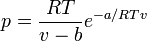$p = \frac{RT}{v-b} e^{-a/RTv}$

where (Eq. 8 in ):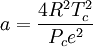$a = \frac{4R^2T_c^2}{P_ce^2}$

and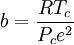$b=\frac{RT_c}{P_ce^2}$

where$p$ is the pressure,$T$ is the temperature and$R$ is the molar gas constant.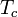$T_c$ is the critical temperature and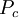$P_c$ is the pressure at the critical point.

Sadus  proposed replacing the repulsive section of the Dieterici equation with the Carnahan-Starling equation of state, which is often used to describe the equation of state of the hard sphere model, resulting in (Eq. 5):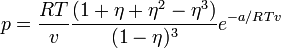$p = \frac{RT}{v} \frac{(1 + \eta + \eta^2 - \eta^3)}{(1-\eta)^3 } e^{-a/RTv}$

where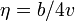$\eta = b/4v$ is the packing fraction.

This equation gives: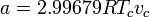$a = 2.99679 R T_c v_c$

and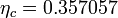$\eta_c = 0.357057$